# Texas Go Math Grade 3 Lesson 14.6 Answer Key Real World Relationships

Refer to our Texas Go Math Grade 3 Answer Key Pdf to score good marks in the exams. Test yourself by practicing the problems from Texas Go Math Grade 3 Lesson 14.6 Answer Key Real World Relationships.

## Texas Go Math Grade 3 Lesson 14.6 Answer Key Real World Relationships

Unlock the Problem

The outdoor club is planning a camping trip. Each camper will need a flashlight. One flashlight uses 4 batteries. How many batteries are needed for 8 flashlights?

You can describe a pattern in a table.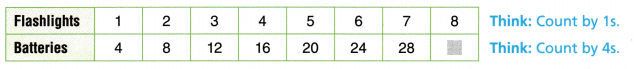One Way Describe a pattern across the rows.

Check that your pattern will work for all the numbers in the table.

STEP 1:
Look for a pattern to complete the table. As you look across the rows, you can see that the number of batteries increases by 4 for each flashlight.
So, for every flashlight add __________ batteries.

STEP 2:
Use the pattern to find the number of batteries in 8 flashlights.
Add __________ to 28 batteries. 28 + 4 = _________
So, ___________ batteries are needed for 8 flashlights.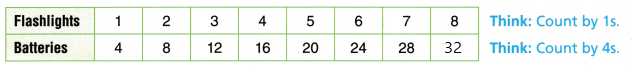Add 4 to 28 batteries. 28 + 4 = 32

So, 32 batteries are needed for 8 flashlights

Another Way Describe a pattern in the columns.

STEP 1:
Look for a pattern by comparing the columns in the table. You can multiply the number of flashlights by 4 to find the number of batteries that are needed.

STEP 2:
Use the pattern to find how many batteries are needed for 8 flashlights.
8 × 4 = ___________
8 × 4 = 32 batteries are needed for 8 flashlights.

Math Talk
Mathematical Processes

Why is it important to know how many batteries are needed for 1 flashlight?

Explanation: If we know 1 flashlight need how many batteries then we can find the pattern

Share and Show

Question 1.
Describe a pattern for the table. Then write a sentence about the cost of 4 packs of batteries.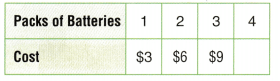Answer: 12$Explanation: To complete the table. As you look across the rows, you can see that the number of batteries increases by 3 for each flashlight. So, for every flashlight add 3 batteries. It costs 12$ for 4 packs of batteries

Describe a pattern for the table. Then complete the table.

Question 2.Explanation:
To complete the table. As you look across the rows, you can see that the number of tents increases by 1 and the tenths are multiplied with 2
9 × 2 = 18
10 × 2 = 20

Question 3.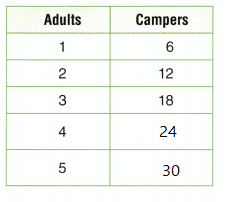Explanation:
To complete the table. As you look across the rows, you can see that the number of adults increases by 1 for each row multiplied with 6 for campers
So, for every adult multiply with 6
4×6=24
5×6=30

Question 4.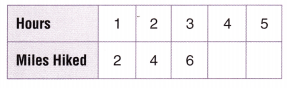Explanation:
To complete the table. As you look across the rows, you can see that the number of miles hiked increases by 2 for each hour
So, for every hour add 2 batteries
6+2=8,
8+2=10

Question 5.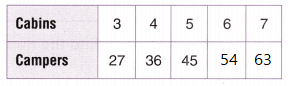Explanation:
To complete the table. As you look across the rows, you can see that the number of Cabins increases by 1 and multiplied 9 to the each cabin
6 x 9 = 54
7 x 9 = 63

Question 6.
Describe a pattern for the table at the right, and complete the table. Then complete each statement below.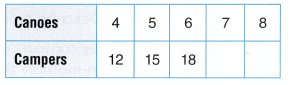__________ canoes have 12 campers.
__________ canoes have 21 campers.

5 canoes have __________ campers.
8 canoes have __________ campers.Explanation:

4 canoes have 12 campers.
7 canoes have 21 campers.

5 canoes have 15 campers.
8 canoes have 24 campers

Problem Solving

Use the pictograph for 7-9.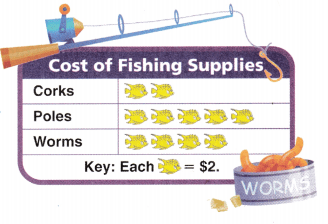Question 7.
Jena bought 3 fishing poles. How much money did she spend?
Explanation:
She spend $6 On three fishing poles each fishing pole =$2

Question 8.
Multi-Step Noah bought 1 fishing pole, 2 corks, and 1 carton of worms. What was the total cost?
Explanation:
1 fishing pole, = 2
2 corks, and 4
1 carton of worms. = 2
The total cost was $8 Question 9. Write Math Ryan bought 8 corks. Explain how you can use the Commutative Property to find the cost. Answer: 16$
Explanation;
1 cork = 2$8 Corks x 2$ = 16$4 + 4 = 8 a commutative property states that if the position of integers are moved around or interchanged while performing addition or multiplication . Question 10. H.O.T. Representations Students made a craft project at camp. They used 2 small pine cone patterns and 1 large pine cone pattern. Complete the table to find how many patterns were used for the different numbers of projects.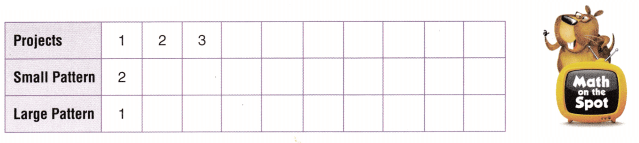Answer:Explanation: For small cane pattern Each number is hiked with 2 For large cane pattern each number is self multiplied with the project numbers Question 11. H.O.T. Multi-Step The cost to rent a raft is$7 per person. A raft can hold up to 6 people. There is a $3 launch fee per raft. What is the total cost for a group of 6? Explain. Answer:$10
Explanation:
According to this sentence
The cost to rent a raft is $7 per person. A raft can hold up to 6 people. There is a$3 launch fee per raft.
7 + 3 = $10$10 is the total cost for a group of 6.

Fill in the bubble for the correct answer choice.

Question 12.
Greg has orchids growing in his garden. Each orchid has 6 blooms. Which of the following describes a pattern in the table?(B) Multiply by 5.
(C) Multiply by 2.
Explanation:
Add 6 describes the pattern in the table.

Question 13.
A chef uses eggs to make omelettes as shown in the table at the right. Which statement below is true?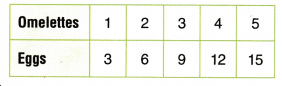(A) The chef uses 2 eggs to make 6 omelettes.
(B) The chef uses 12 eggs to make 3 omelettes.
(C) The chef uses 9 eggs to make 3 omelettes.
(D) The chef uses 4 eggs to make 12 omelettes.
Explanation:
The chef uses 9 eggs to make 3 omelettes. is true

Question 14.
Multi-Step A group of students and adults are going on a field trip in vans. In each van, there will be 8 students and 2 adults. How many people will be in 4 vans?
(A) 40
(B) 10
(C) 14
(D) 32
Explanation:
A group of students and adults are going on a field trip in vans.
In each van, there will be 8 students and 2 adults.
8 + 2 = 10
4 x 10 = 40
40 people will be in 4 vans

Texas Test Prep

Question 15.
Which of the following describes a pattern in the table?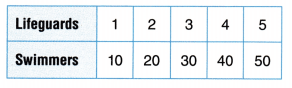(B) Multiply by 2.
(C) Subtract 9.
(D) Multiply by 10.
Explanation:
Multiply by 10 Describes the pattern in the table

### Texas Go Math Grade 3 Lesson 14.6 Homework and Practice Answer Key

Describe a pattern for the table. Then complete the table.

Question 1.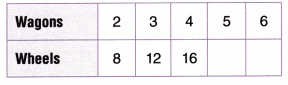Explanation:
Multiply by 4 Describes the pattern in the table
5 x 4 = 20
6 x 4 = 24

Question 2.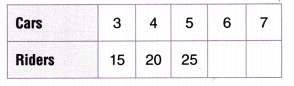Explanation:
Multiply by 5 Describes the pattern in the table
6 x 5 = 30
7 x 5 = 35

Question 3.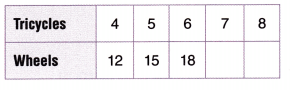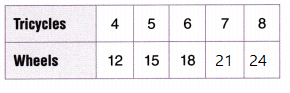Explanation:
Multiply by 3 Describes the pattern in the table
7 x 3 = 21
8 x 3 = 24

Question 4.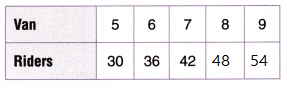Explanation:
Multiply by 6 Describes the pattern in the table
8 x 6 = 48
9 x 6 = 54

Problem Solving

Use the pictograph for 5-7.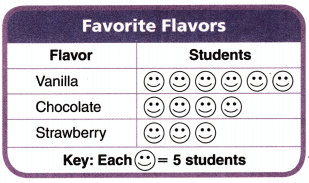Question 5.
How many students chose vanilla as their favorite flavor?
Explanation:
6 x 5 = 30 students chose vanilla as their favorite flavor
With help of above table and the key given

Question 6.
Two more students voted for strawberry. Now how many students chose strawberry as their favorite flavor?
Explanation:
3 + 2 = 5
5 x 5 = 25 students chose strawberry as their favorite flavor
With help of above table and the key given

Question 7.
How many more students need to vote for chocolate to tie for votes with vanilla?
Explanation:
4 + 2 = 6 more students need to vote for chocolate to tie for votes with vanilla
With help of above table and the key given

Lesson Check

Question 8.
Meg puts 5 tails on 1 kite. How many tails does she need for 5 kites?(A) 16
(B) 20
(C) 30
(D) 25Explanation:
Meg puts 5 tails on 1 kite.
25 tails  she need for 5 kites.

Question 9.
Shanaz puts 3 dimes in her bank each day. How many dimes will she have on day 4?(A) 15
(B) 12
(C) 9
(D) 7Explanation:
Shanaz puts 3 dimes in her bank each day.
12  dimes  she should have on day 4.

Question 10.
Which describes a pattern in the table?(A) Subtract 6.
(B) Multiply by 5.
(D) Multiply by 2.
Explanation:
Add  by 6 Describes the pattern in the table

Question 11.
Which describes a pattern in the table?(C) Multiply by 6.
(D) Multiply by 5.
Explanation:
Add by 4 Describes the pattern in the table

Question 12.
Multi-Step Isaac uses 4 red beads and 3 blue beads to make a belt. How many beads will Isaac use to make 4 belts?
(A) 48
(B) 12
(C) 28
(D) 16
Explanation:
Isaac uses 4 red beads and 3 blue beads to make a belt.
4 x 3 = 12
12 x 4 = 48
48 beads will Isaac use to make 4 belts.

Question 13.
Multi-Step Corey uses 5 yellow tiles and 4 green tiles to make a design. How many tiles will he need to repeat the design 5 times?
(A) 45
(B) 9
(C) 25
(D) 20